## Bernoulli's Equation

The differential equation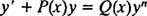is known as Bernoulli's equation. If n = 0, Bernoulli's equation reduces immediately to the standard form first‐order linear equation:If n = 1, the equation can also be written as a linear equation:However, if n is not 0 or 1, then Bernoulli's equation is not linear. Nevertheless, it can be transformed into a linear equation by first multiplying through by y n ,and then introducing the substitutions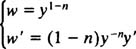The equation above then becomeswhich is linear in w (since n ≠ 1).

Example 1: Solve the equationNote that this fits the form of the Bernoulli equation with n = 3. Therefore, the first step in solving it is to multiply through by y n = y −3: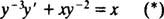Now for the substitutions; the equations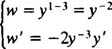transform (*) into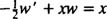or, in standard form,Notice that the substitutions were successful in transforming the Bernoulli equation into a linear equation (just as they were designed to be). To solve the resulting linear equation, first determine the integrating factor: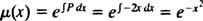Multiplying (**) through the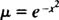yields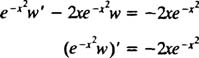And an integration gives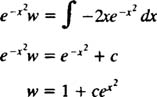The final step is simply to undo the substitution w = y −2. The solution to the original differential equation is therefore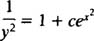Back to Top
A18ACD436D5A3997E3DA2573E3FD792A